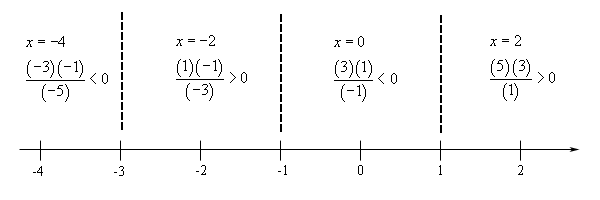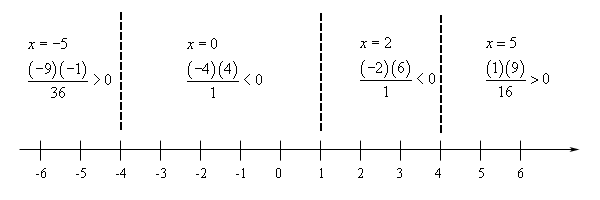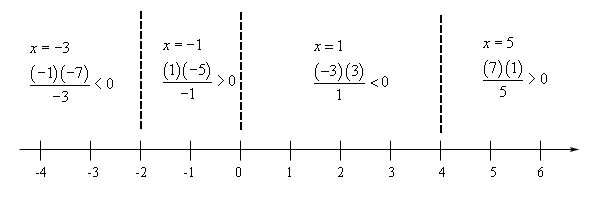Paul's Online Notes
Home / Algebra / Solving Equations and Inequalities / Rational Inequalities
Show Mobile Notice Show All Notes Hide All Notes
Mobile Notice
You appear to be on a device with a "narrow" screen width (i.e. you are probably on a mobile phone). Due to the nature of the mathematics on this site it is best views in landscape mode. If your device is not in landscape mode many of the equations will run off the side of your device (should be able to scroll to see them) and some of the menu items will be cut off due to the narrow screen width.

### Section 2.13 : Rational Inequalities

In this section we will solve inequalities that involve rational expressions. The process for solving rational inequalities is nearly identical to the process for solving polynomial inequalities with a few minor differences.

Let’s just jump straight into some examples.

Example 1 Solve $$\displaystyle \frac{{x + 1}}{{x - 5}} \le 0$$.
Show Solution

Before we get into solving these we need to point out that these DON’T solve in the same way that we’ve solve equations that contained rational expressions. With equations the first thing that we always did was clear out the denominators by multiplying by the least common denominator. That won’t work with these however.

Since we don’t know the value of $$x$$ we can’t multiply both sides by anything that contains an $$x$$. Recall that if we multiply both sides of an inequality by a negative number we will need to switch the direction of the inequality. However, since we don’t know the value of $$x$$ we don’t know if the denominator is positive or negative and so we won’t know if we need to switch the direction of the inequality or not. In fact, to make matters worse, the denominator will be both positive and negative for values of $$x$$ in the solution and so that will create real problems.

So, we need to leave the rational expression in the inequality.

Now, the basic process here is the same as with polynomial inequalities. The first step is to get a zero on one side and write the other side as a single rational inequality. This has already been done for us here.

The next step is to factor the numerator and denominator as much as possible. Again, this has already been done for us in this case.

The next step is to determine where both the numerator and the denominator are zero. In this case these values are.

${\mbox{numerator : }}x = - 1\hspace{0.5in}{\mbox{denominator : }}x = 5$

Now, we need these numbers for a couple of reasons. First, just like with polynomial inequalities these are the only numbers where the rational expression may change sign. So, we’ll build a number line using these points to define ranges out of which to pick test points just like we did with polynomial inequalities.

There is another reason for needing the value of $$x$$ that make the denominator zero however. No matter what else is going on here we do have a rational expression and that means we need to avoid division by zero and so knowing where the denominator is zero will give us the values of $$x$$ to avoid for this.

Here is the number line for this inequality.So, we need regions that make the rational expression negative. That means the middle region. Also, since we’ve got an “or equal to” part in the inequality we also need to include where the inequality is zero, so this means we include $$x = - 1$$. Notice that we will also need to avoid $$x = 5$$ since that gives division by zero.

The solution for this inequality is,

$- 1 \le x < 5\hspace{0.25in}\left[ { - 1,5} \right)$
Example 2 Solve $$\displaystyle \frac{{{x^2} + 4x + 3}}{{x - 1}} > 0$$.
Show Solution

We’ve got zero on one side so let’s first factor the numerator and determine where the numerator and denominator are both zero.

$\frac{{\left( {x + 1} \right)\left( {x + 3} \right)}}{{x - 1}} > 0$ ${\mbox{numerator : }}x = - 1,\,\,\,\,x = - 3\hspace{0.5in}{\mbox{denominator : }}x = 1$

Here is the number line for this one.In the problem we are after values of $$x$$ that make the inequality strictly positive and so that looks like the second and fourth region and we won’t include any of the endpoints here. The solution is then,

$\begin{array}{c} - 3 < x < - 1\hspace{0.25in}{\mbox{and}}\hspace{0.25in}1 < x < \infty \\\left( { - 3, - 1} \right)\hspace{0.25in}{\mbox{and}}\hspace{0.25in}\,\,\,\,\left( {1,\infty } \right)\end{array}$
Example 3 Solve $$\displaystyle \frac{{{x^2} - 16}}{{{{\left( {x - 1} \right)}^2}}} < 0$$.
Show Solution

There really isn’t too much to this example. We’ll first need to factor the numerator and then determine where the numerator and denominator are zero.

$\frac{{\left( {x - 4} \right)\left( {x + 4} \right)}}{{{{\left( {x - 1} \right)}^2}}} < 0$ ${\mbox{numerator : }}x = - 4,\,\,\,\,x = 4\hspace{0.5in}{\mbox{denominator : }}x = 1$

The number line for this problem is,So, as with the polynomial inequalities we can not just assume that the regions will always alternate in sign. Also, note that while the middle two regions do give negative values in the rational expression we need to avoid $$x = 1$$ to make sure we don’t get division by zero. This means that we will have to write the answer as two inequalities and/or intervals.

$\begin{array}{c} - 4 < x < 1\hspace{0.25in}{\mbox{and}}\hspace{0.25in}1 < x < 4\\ \left( { - 4,1} \right)\hspace{0.25in}{\mbox{and}}\hspace{0.25in}\,\,\,\,\left( {1,4} \right)\end{array}$

Once again, it’s important to note that we really do need to test each region and not just assume that the regions will alternate in sign.

Next, we need to take a look at some examples that don’t already have a zero on one side of the inequality.

Example 4 Solve $$\displaystyle \frac{{3x + 1}}{{x + 4}} \ge 1$$.
Show Solution

The first thing that we need to do here is subtract 1 from both sides and then get everything into a single rational expression.

\begin{align*}\frac{{3x + 1}}{{x + 4}} - 1 & \ge 0\\ \frac{{3x + 1}}{{x + 4}} - \frac{{x + 4}}{{x + 4}} & \ge 0\\ \frac{{3x + 1 - \left( {x + 4} \right)}}{{x + 4}} & \ge 0\\ \frac{{2x - 3}}{{x + 4}} & \ge 0\end{align*}

In this case there is no factoring to do so we can go straight to identifying where the numerator and denominator are zero.

${\mbox{numerator : }}x = \frac{3}{2}\hspace{0.5in}{\mbox{denominator : }}x = - 4$

Here is the number line for this problem.Okay, we want values of $$x$$ that give positive and/or zero in the rational expression. This looks like the outer two regions as well as $$x = \frac{3}{2}$$. As with the first example we will need to avoid $$x = - 4$$ since that will give a division by zero error.

The solution for this problem is then,

$\begin{array}{c} \displaystyle - \infty < x < - 4\hspace{0.25in}{\mbox{and}}\hspace{0.25in}\frac{3}{2} \le x < \infty \\ \displaystyle \left( { - \infty , - 4} \right)\hspace{0.25in}{\mbox{and}}\hspace{0.25in}\,\,\,\left[ {\frac{3}{2},\infty } \right)\end{array}$
Example 5 Solve $$\displaystyle \frac{{x - 8}}{x} \le 3 - x$$.
Show Solution

So, again, the first thing to do is to get a zero on one side and then get everything into a single rational expression.

\begin{align*}\frac{{x - 8}}{x} + x - 3 & \le 0\\ \frac{{x - 8}}{x} + \frac{{x\left( {x - 3} \right)}}{x} & \le 0\\ \frac{{x - 8 + {x^2} - 3x}}{x} & \le 0\\ \frac{{{x^2} - 2x - 8}}{x} & \le 0\\ \frac{{\left( {x - 4} \right)\left( {x + 2} \right)}}{x} & \le 0\end{align*}

We also factored the numerator above so we can now determine where the numerator and denominator are zero.

${\mbox{numerator : }}x = - 2,\,\,\,\,x = 4\hspace{0.5in}{\mbox{denominator : }}x = 0$

Here is the number line for this problem.The solution for this inequality is then,

$\begin{array}{c} - \infty < x \le - 2\hspace{0.25in}{\mbox{and}}\hspace{0.25in}0 < x \le 4\\ \left( { - \infty , - 2} \right]\hspace{0.25in}{\mbox{and}}\hspace{0.25in}\,\,\,\,\left( {0,4} \right]\end{array}$